# Electrical characteristics of bipolar transistors

The following is an example of the electrical characteristics table for an NPN transistor.

Electrical Characteristics
Characteristic Symbol Test Conditions Min Typ Max Unit
1. Collector cut-off current ICBO VCB = 60 V, IE = 0 0.1 μA
2. Emitter cut-off current IEBO VEB = 5 V, IC = 0 0.1 μA
3. DC current gain hFE(1)(Note) VCE = 6 V, IC = 2mA 70 700
hFE(2) VCE = 6 V, IC = 150 mA 25 100
4. Collector-emitter saturation voltage VCE(SAT) IC = 100 mA, IB = 10 mA 0.1 0.25 V
5. Base-emitter saturation voltage VBE(SAT) IC = 100 mA, IB = 10 mA 1.0 V
Transition frequency fT VCE = 10 V, IC = 1 mA 80 MHz
Collector output capacitance Cob VCB = 10 V, IE = 0, f = 1MHz 2.0 3.5 pF
Resistor in series with the base rbb' VCE = 10 V, IE = -1mA, f = 30MHz 50 Ω
Noise figure NF VCE = 6 V, IC = 0.1 mA, f = 1 kHz, RG = 10 kΩ 1 10 dB
• Note: hFE(1) classification  O: 70 to 140, Y: 120 to 240, GR: 200 to 400, BL: 350 to 700
1. Collector cut-off current

ICBO measurement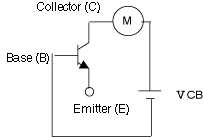2. Emitter cut-off current

IEBO measurement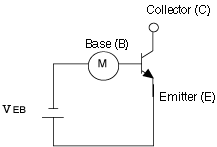3. DC current gain

DC current gain hFE measurement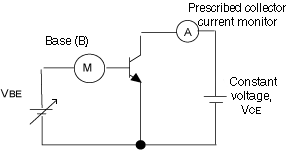Increase the base-emitter voltage, VBE, until the collector current, IC, reaches the specified value. Adjust VBE to keep IC constant and measure the base current, IB. Calculate the DC current gain, hFE, as shown below:
hFE=IC/IB

Example of a DC current gain (hFE) curve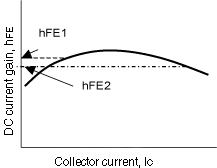4. Collector-emitter saturation voltage

Measurement of collector-emitter saturation voltage, VCE(sat)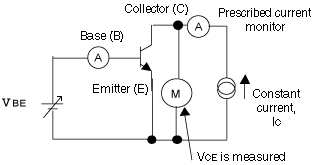Apply the specified constant collector current, IC, to increase the base-emitter voltage, VBE, until the base current, IB, reaches the specified value. Adjust IC to keep IB constant and measure the collector-emitter voltage.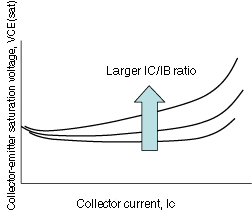5. Base-emitter saturation voltage

Measurement of base-emitter saturation voltage, VBE(sat)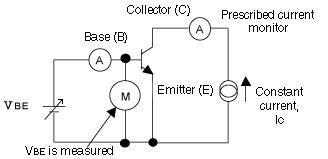Apply the specified constant collector current, IC, to increase the base-emitter voltage, VBE, until the base current, IB, reaches the specified value. Adjust IC to keep IB constant and measure the base-emitter voltage.

Note: Keep the open-circuit voltage of the constant-current source below the collector-emitter breakdown voltage.

Example of collector-emitter saturation voltage (VCE(sat)) curves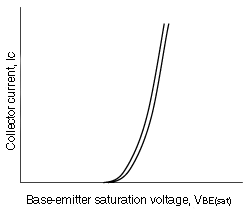A new window will open